## Hilbert Function

Letbe a collection ofdistinct points. Then the number of conditions imposed by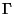on forms of degreeis called the Hilbert functionof. If curvesandof degreesandmeet in a collectionofpoints, then for any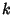, the number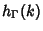of conditions imposed byon forms of degreeis independent ofandand is given by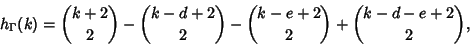where the Binomial Coefficientis taken as 0 if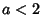(Cayley 1843).

References

Eisenbud, D.; Green, M.; and Harris, J. Cayley-Bacharach Theorems and Conjectures.'' Bull. Amer. Math. Soc. 33, 295-324, 1996.